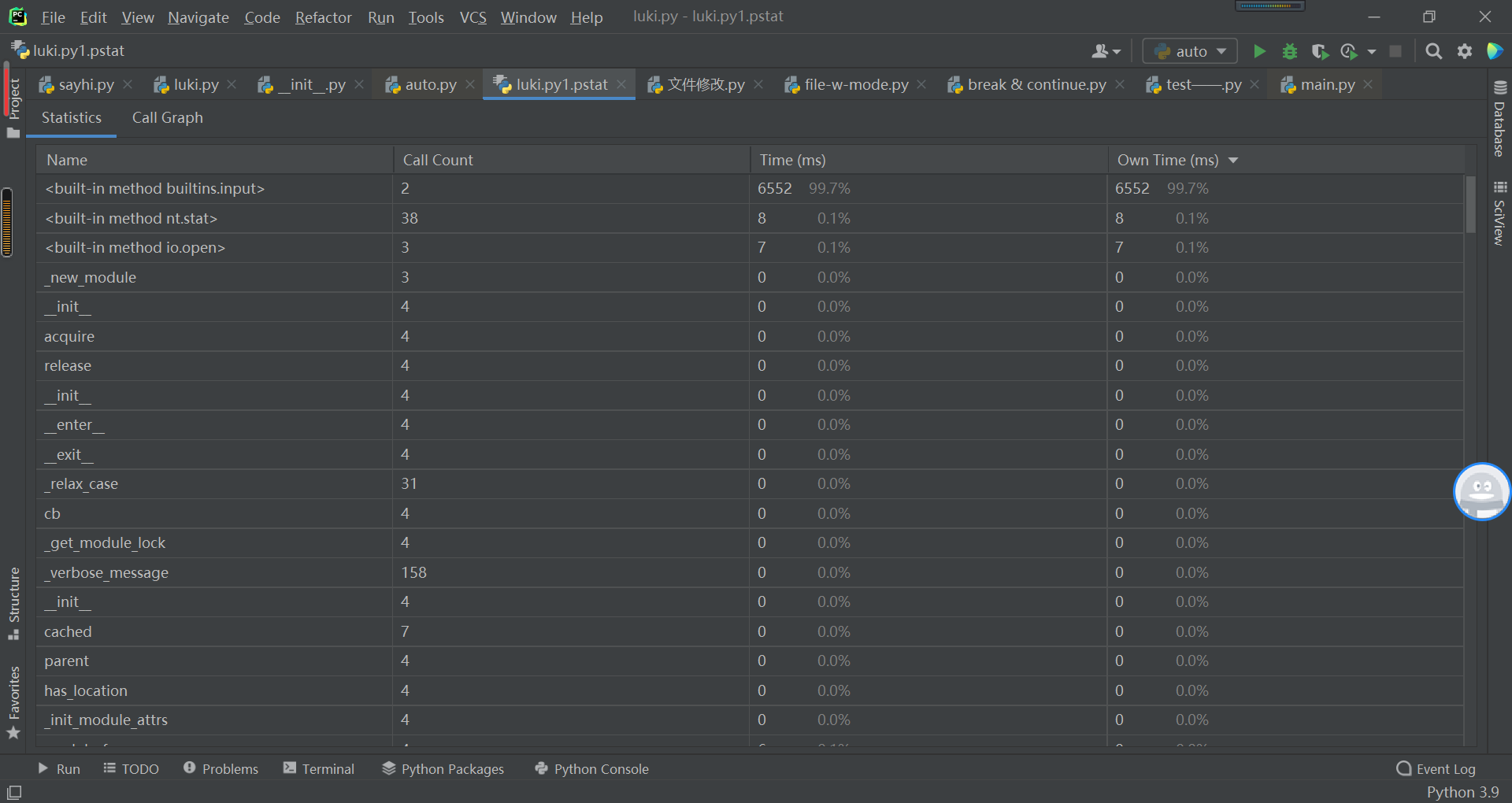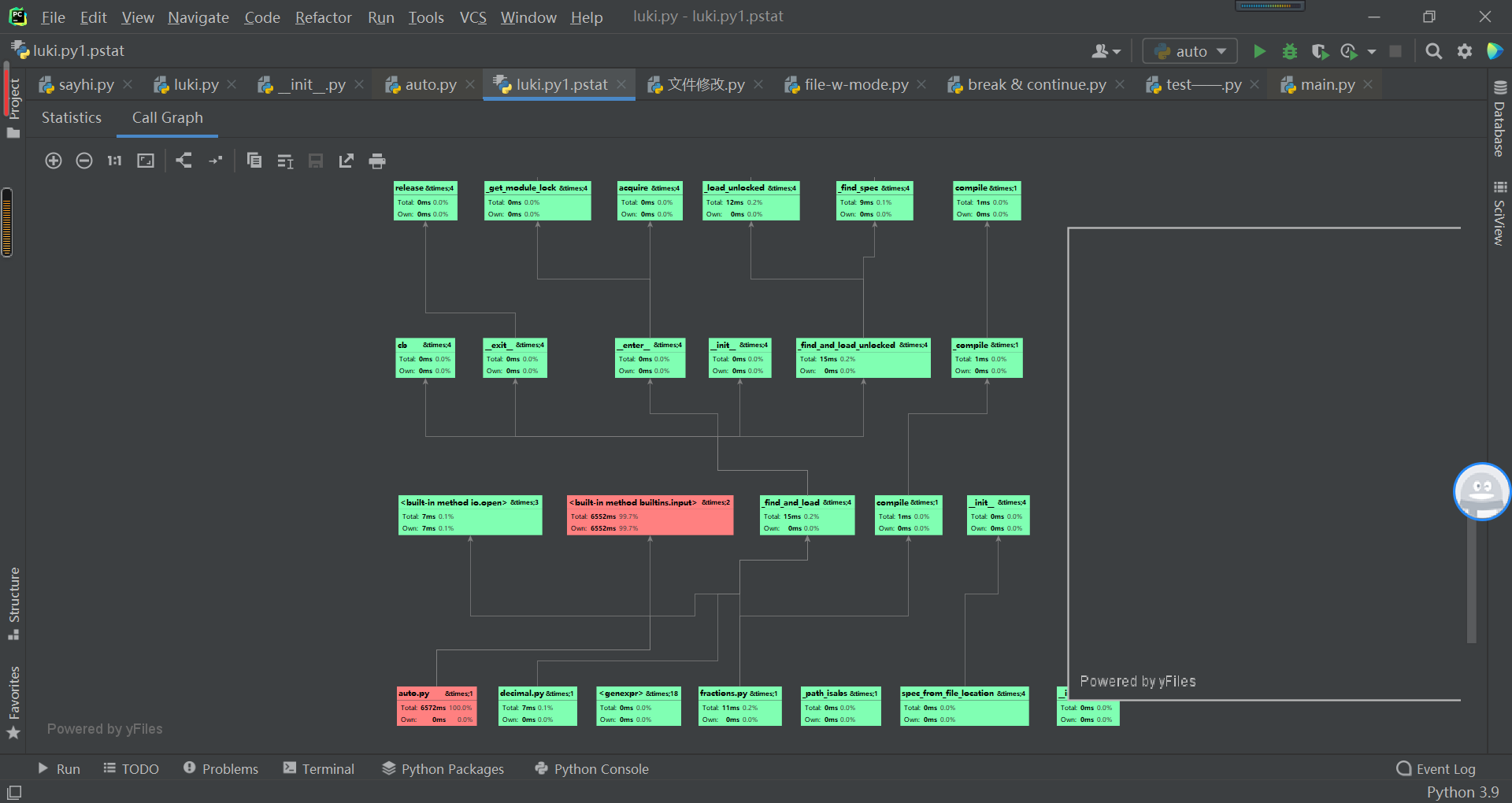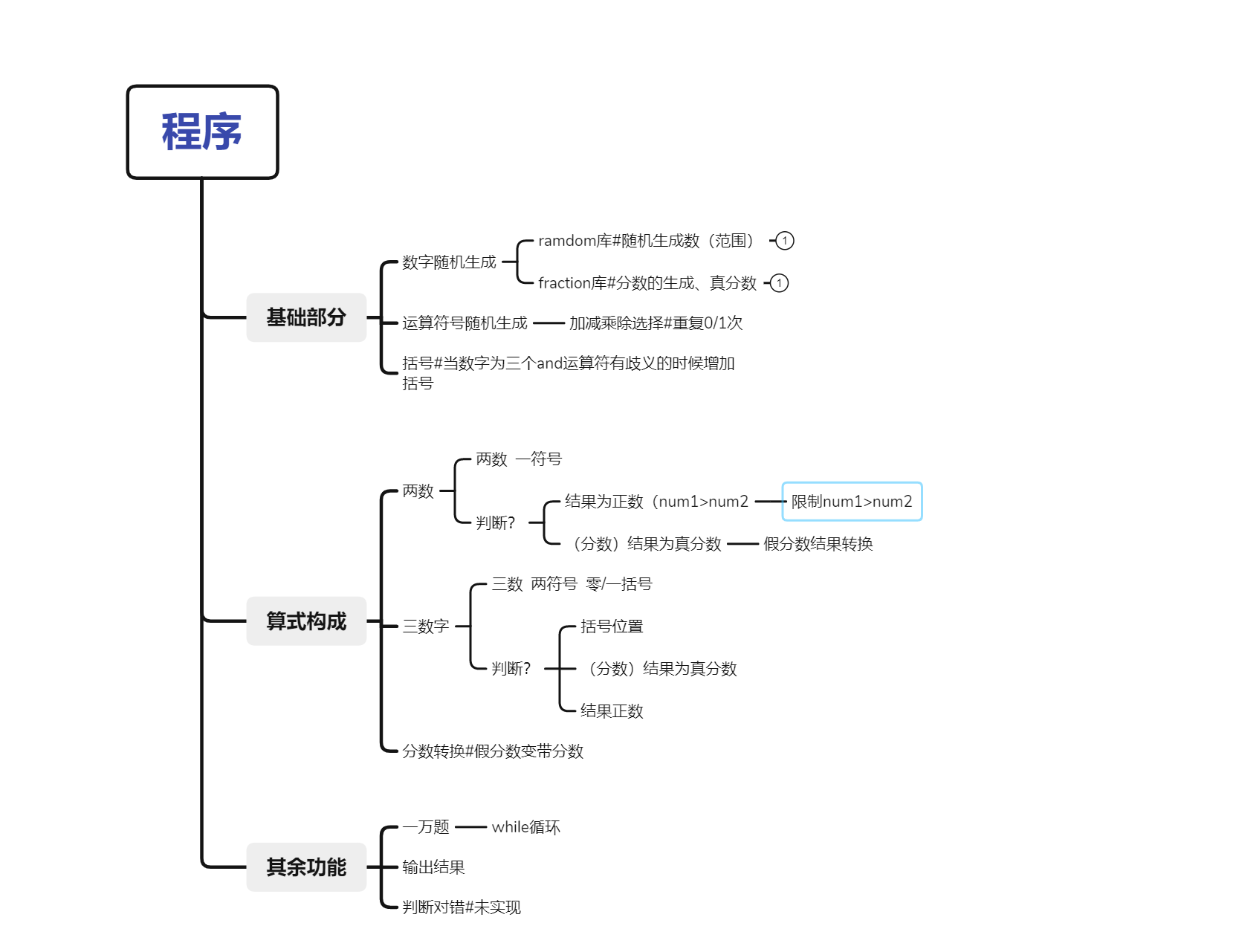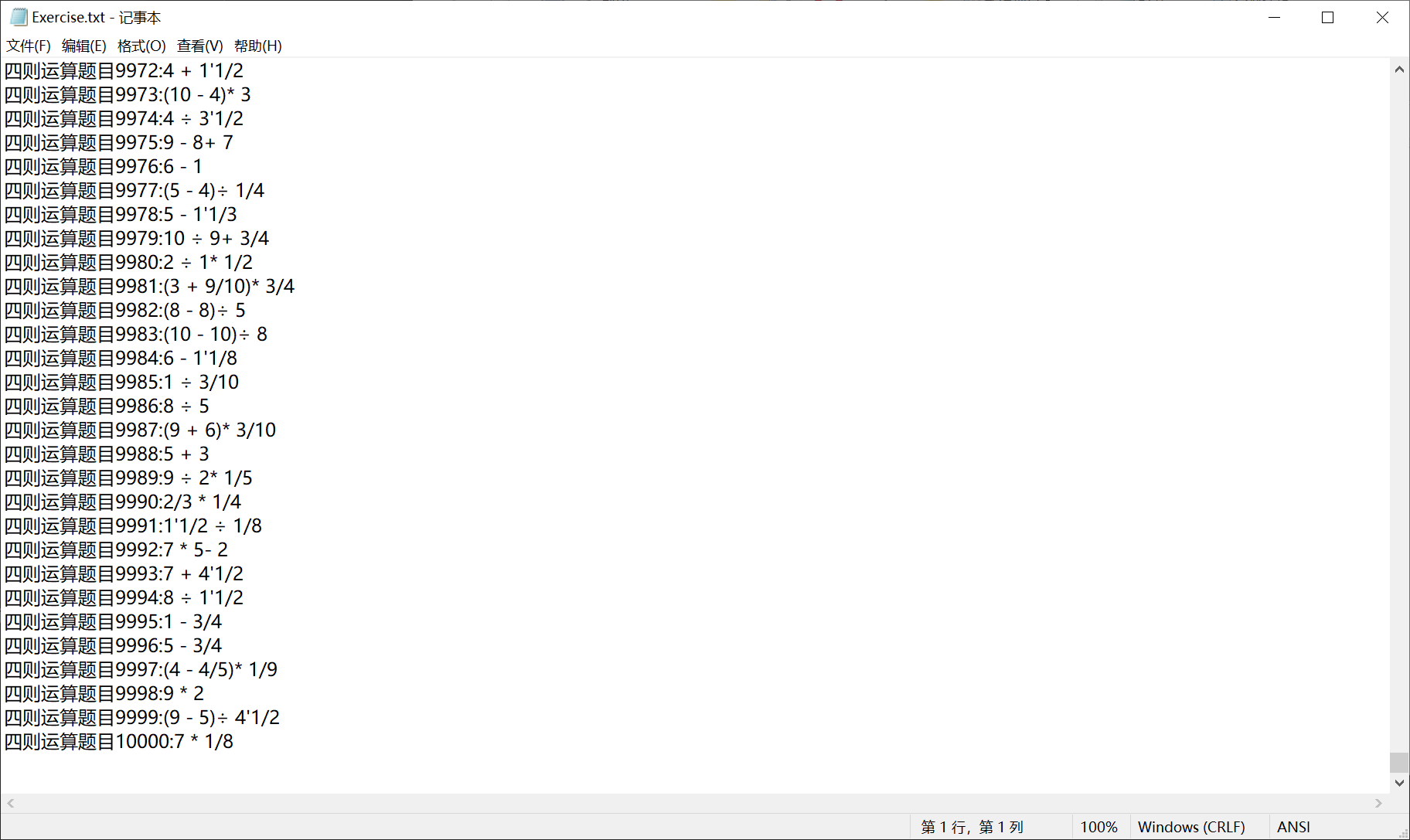# 第二次编程作业（结对编程）

| 这个作业属于哪个课程 | 19计科国际班的软工 |

| 这个作业要求在哪里| 作业要求|

| 这个作业的目标 | 实现一个自动生成小学四则运算题目的命令行程序 |

| 成员 | 学号 |

| 邓子昊 | 3119009459 |

| 何梓健 | 3119009460 |

GitHub地址：github地址

### PSP表格

||||||||||||||

PSP2.1 Personal Software Process Stages 预估耗时（分钟） 实际耗时（分钟）
Planning 计划 120 150
Estimate · 估计这个任务需要多少时间 30 35
Analysis · 需求分析 (包括学习新技术) 20 40
Design Spec · 生成设计文档 10 15
Design Review · 设计复审 (和同事审核设计文档) 30 50
Coding Standard · 代码规范 (为目前的开发制定合适的规范) 70 70
Design · 具体设计 150 180
Coding · 具体编码 300 280
Code Review · 代码复审 30 90
Test · 测试（自我测试，修改代码，提交修改） 60 90
Reporting 报告 120 120
Test Report · 测试报告 10 10
Size Measurement · 计算工作量 10 10
Postmortem & Process Improvement Plan · 事后总结, 并提出过程改进计划 30 30

### 效能分析### 设计实现过程### 代码说明i = random.choice(['整', '分'])
if i == '整':
num1 = random.randint(1, n)
num_int = Fraction(num1, 1)
list1.append(num_int)             #出整数
elif i == '分':
num1 = random.randint(1, n)       #分子
num2 = random.randint(1, n)       #分母
# 判断max公约数
mi = max(num1, num2)
ni = min(num1, num2)
r = mi % ni
while r != 0:
mi = ni
ni = r
r = mi % ni
if ni != 1:
num1 //= ni
num2 //= ni
if num2 == 0 or num1 % num2 == 0:
continue
if num1 >= num2:
create_fakefrac(num1, num2)
num_frac = Fraction(num1, num2)          #出真分数
list1.append(num_frac)


def create_fakefrac(x, y):
num_inte = x//y        #商
num_fra = x%y       #余数
if num_fra == 0:
return Fraction(x,y)
else:
if num_inte == 0:
return Fraction(x,y)
else:
str = f"{num_inte}'{num_fra}/{y}"
return str


def output_string_2(y):
if (y.numerator > y.denominator):
res3 = create_fakefrac(y.numerator, y.denominator)
else:
res3 = y
e.write(("+ %s" % res3) + "\n")
def minus2():
if (y.numerator > y.denominator):
res3 = create_fakefrac(y.numerator, y.denominator)
else:
res3 = y
e.write(("- %s" % res3) + "\n")
def mult2():
if (y.numerator > y.denominator):
res3 = create_fakefrac(y.numerator, y.denominator)
else:
res3 = y
e.write(("* %s" % res3) + "\n")
def divi2():
if (y.numerator > y.denominator):
res3 = create_fakefrac(y.numerator, y.denominator)
else:
res3 = y
e.write(("÷ %s" % res3) + "\n")
switch = {
2 :minus2,
3 :mult2,
4 :divi2
}


### 测试运行### 项目小结

#### 何梓健的感想：

这次结对编程作业让我体会到了两个人的编程其实是一件挺让人感慨的事，我和邓同学基本上都担任了驾驶员和领航员，而且在这两种身份上花费了差不多的时间。我负责输出这方面的工作，邓同学负责整体程序的组成。两个人从开始的磨合，互相帮助互相监督，不能只按照自己的代码规范写，也要考虑到同伴的阅读、检查代码的感受。


#### 邓子昊的感想

在这次作业中，我第一次体验到了结对合作的模式，也深刻地感觉到了两个人合作和一个人合作的不一样。单人的时候有关代码规范，注释的部分经常就会自己随便决定，但是双人的话需要保障自己的队友能够看得懂并且理解，这就不能随便来了。碰到比较多的问题还有元素的定义。有时候两个人定义了相同的元素却用来做不同的功能，直到最后检查出才发现。如果一开始能够制定规范将会为工作减少不少困难。学到了很多东西，希望下次还有机会。

posted @ 2021-10-12 00:44  LucianoRB  阅读(12)  评论(0编辑  收藏  举报